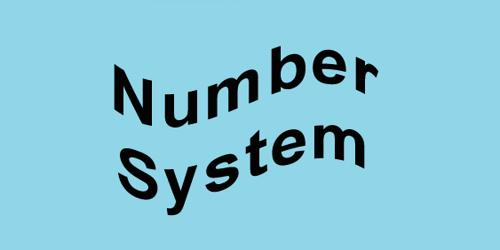Mathematics

# Base of Number SystemThe base of Number System

The base of a number system is the total number of fundamental sign used in that system. For example, there are ten fundamental signs in the decimal system. For example, 0, 1, 2, 3, 4, 5, 6, 7, 8, 9. So, its base is 10. Depending on the base of the number system positional number can be of different types. In the digital circuit, four types of mathematical systems are used. The process of writing a number is called number system. A number system is a collection of various symbols which are called digits. In mathematical numeral systems, the radix or base is the number of unique digits, including the digit zero, used to represent numbers in a positional numeral system. For example, for the decimal system (the most common system in use today) the radix is ten because it uses the ten digits from 0 through 9. These are:

1. decimal or base 10 number system,
2. binary or base, 2 number system,
3. octal or base 8 number system,
4. hexadecimal or base 16 number system etc.